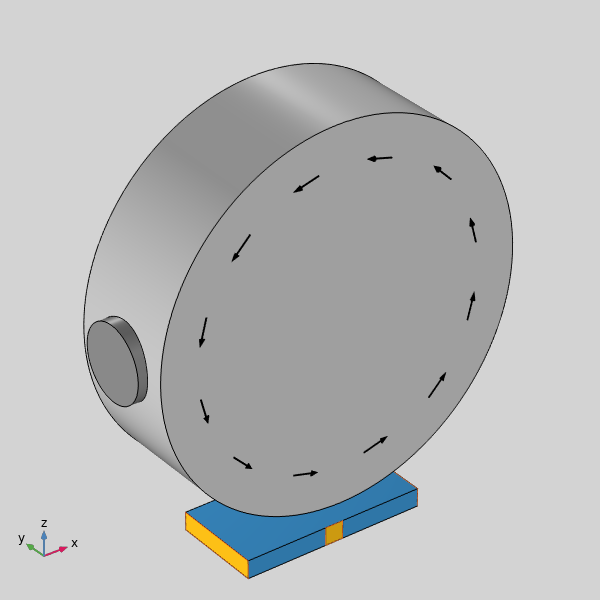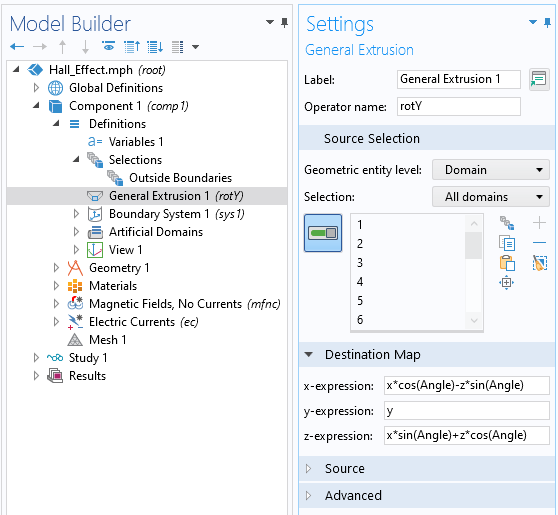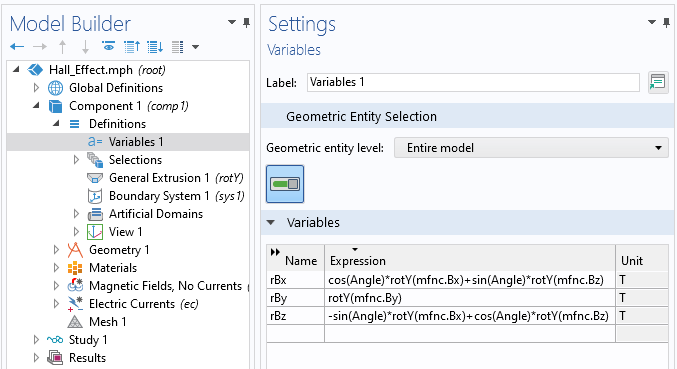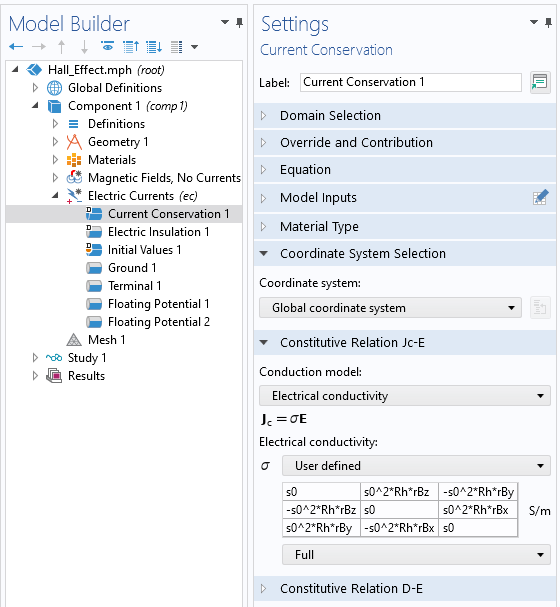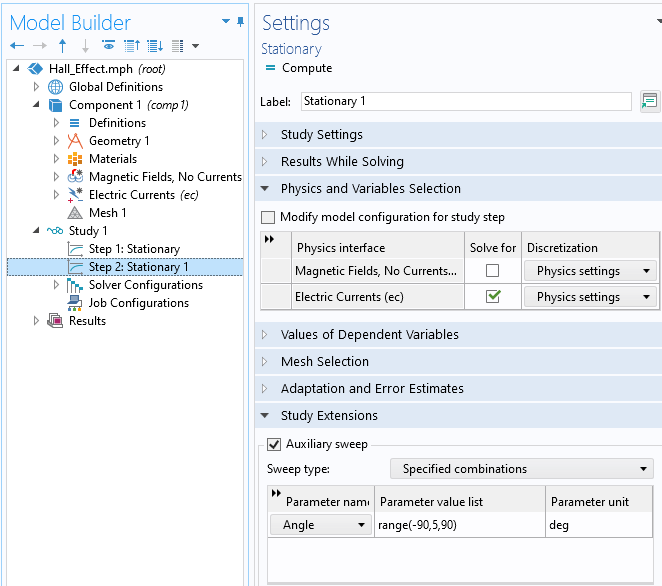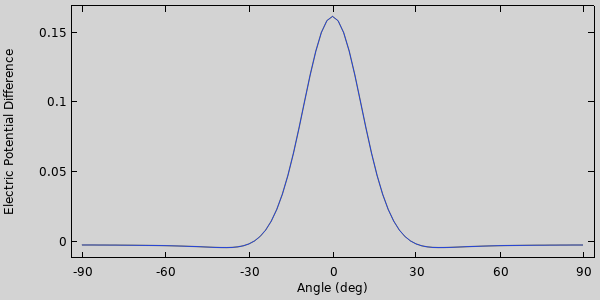# 如何使用 COMSOL Multiphysics® 模拟霍尔效应传感器

2021年 3月 11日

### 霍尔效应传感器的基本工作原理

\mathbf{F}=q \left ( \mathbf{E+ v \times B} \right)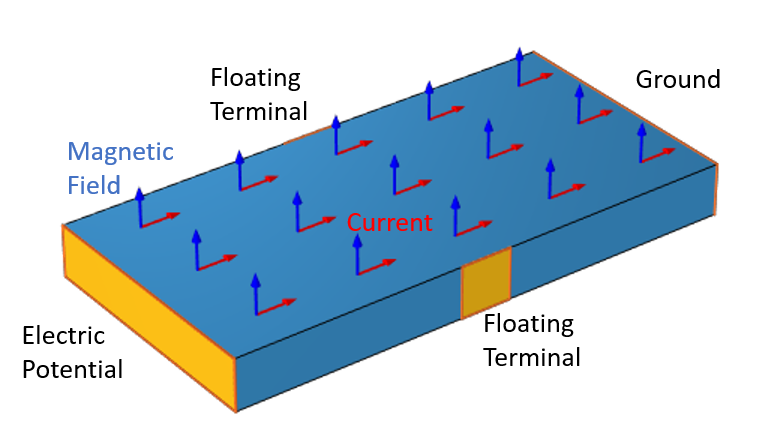\mathbf{J}=\sigma \left( \mathbf{E}+ \sigma R_H \left( \mathbf{E \times B} \right) \right)

\begin{Bmatrix}J_x \\ J_y \\ J_z\end{Bmatrix}=\begin{bmatrix}\sigma & \sigma^2R_H B_z & -\sigma^2 R_H B_y\\-\sigma^2R_H B_z & \sigma & \sigma^2 R_H B_x\\\sigma^2 R_H B_y & -\sigma^2R_H B_x & \sigma\end{bmatrix} \begin{Bmatrix}E_x \\ E_y \\ E_z\end{Bmatrix}

\nabla \times \mathbf{E}=-\frac{\partial \mathbf{B} }{\partial t}

### 在 COMSOL Multiphysics® 中建立模型